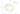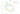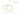## Regularized Logistic Regression

#### Cost function

The cost function for regularized logistic regression is:

$J(\theta) = - \space \frac{1}{m} \displaystyle \sum_{i=1}^m \left[ y^{(i)}\log (h_\theta (x^{(i)})) + (1 - y^{(i)})\log (1 - h_\theta(x^{(i)})) \right] + \frac{\lambda}{2m} \ \sum_{j=1}^n \theta_j^2$

The gradient descent algorithm for regularized logistic regression in terms of hypothesis function $h_\theta(x)$ looks exactly same as that for the regularized linear regression:

\begin{aligned} & \text{Repeat}\ \lbrace \\ & \ \ \ \ \theta_0 := \theta_0 - \alpha\ \frac{1}{m} \sum\limits_{i=1}^{m}\left((h_\theta(x^{(i)}) - y^{(i)}) x^{(i)}_{0}\right) \\ & \ \ \ \ \theta_j := \theta_j - \alpha\ \left[ \frac{1}{m} \sum\limits_{i=1}^{m}\left((h_\theta(x^{(i)}) - y^{(i)}) x^{(i)}_{j}\right) + \frac{\lambda}{m}\theta_j \right] &\ \ \ \ \ \ \ j \in \lbrace 1,2...n\rbrace\\ & \rbrace \end{aligned}

After some manipulation, this can be written as:

\begin{aligned} & \text{Repeat}\ \lbrace \\ & \ \ \ \ \theta_0 := \theta_0 - \alpha\ \frac{1}{m} \sum\limits_{i=1}^{m}\left((h_\theta(x^{(i)}) - y^{(i)}) x^{(i)}_{0}\right) \\ & \ \ \ \ \theta_j := \theta_j \ \Big( 1 - \alpha \ \frac{\lambda}{m} \Big) - \alpha\ \frac{1}{m} \sum\limits_{i=1}^{m}\left((h_\theta(x^{(i)}) - y^{(i)}) x^{(i)}_{j}\right) &\ \ \ \ \ \ j \in \lbrace 1,2...n\rbrace\\ & \rbrace \end{aligned}

Example: Varying $\lambda$ for regularized logistic regressionFigure 1: Training data with decision boundary ($\lambda$ = 1)Figure 2: No regularization (Overfitting) ($\lambda$ = 0)Figure 3: Too much regularization (Underfitting) ($\lambda$ = 100)